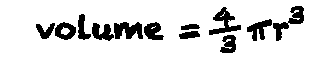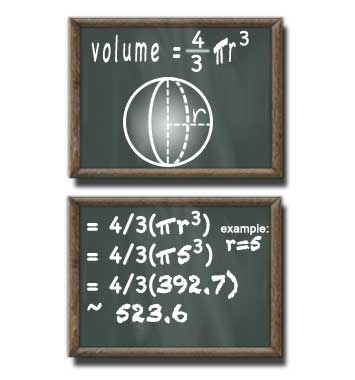# Volume of a Sphere Calculator

## Sphere Volume

#### For Finding Volume of a Sphere

Result:
523.599decimals

A sphere with a radius of 5 units has a volume of 523.599 cubed units.

This calculator and more easy to use calculators waiting at www.KylesCalculators.com### Calculating the Volume of a Sphere:

Volume (denoted 'V') of a sphere with a known radius (denoted 'r') can be calculated using the formula below:

V = 4/3(PI*r3)

In plain english the volume of a sphere can be calculated by taking four-thirds of the product of radius (r) cubed and PI.

You can approximated PI using: 3.14159. If the number you are given for the radius does not have a lot of digits you may use a shorter approximation. If the radius you are given has a lot of digits then you may need to use a longer approximation.

Here is a step-by-step case that illustrates how to find the volume of a sphere with a radius of 5 meters. We'll use 3.14 to approximate PI because the radius we're given only has one digit.

• V = 4/3(pi * r3
• = 4/3(3.14 * 53)
• = 4/3(3.14 * 125)
• = 4/3(392.5)
• = 523.333... meters

### Need help converting volume to other units?

Volume unit converts